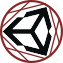#Uninomicon

Documenting the dark corners of the Unity Engine.

ienumerator

# IEnumerator

Notes on the underlying implementation of C# generators (which are used in Unity for coroutines).

You can use SharpLab to view the decompiled C# from a simple generator, to help understand the transformation that is happening. Both .NET and Mono do seem to use the same compiler transformation when turning Generators into anonymous IEnumerator classes. example

## States

Generators are compiled down into a state machine, with states for each `yield return` point. ie:

• 0: The generator has not yet been run.
• 1: The generator has paused on the first yield return.
• 2: The generator has paused on the second yield return.
• -1: The generator has finished, the generator exited early, or the generator threw an exception.

The state value is set in a field `private int <>1__state` on the generated IEnumerator class:

```    [CompilerGenerated]
private sealed class <M>d__0 : IEnumerator<object>, IDisposable, IEnumerator
{
private int <>1__state;

private object <>2__current;

public C <>4__this;
// snipped```

## Lambda function DisplayClass classes are initialized at the start of the function

The following code, in SharpLab decompiles it as:

```using System;
using System.Collections;
public class C {
public IEnumerator M() {
int x = 5;
yield return Test(() => x > 5);
yield return 5;
yield return 5;
yield return 5;
}

public IEnumerator Test(Func<bool> func) {
yield return func();
}
}```
```.. snipped
private bool MoveNext()
{
switch (<>1__state)
{
default:
return false;
case 0:
<>1__state = -1;
<>8__1 = new <>c__DisplayClass0_0();
<>8__1.x = 5;
<>2__current = 5;
<>1__state = 1;
return true;
case 1:
<>1__state = -1;
<>2__current = 5;
<>1__state = 2;
return true;
case 2:
<>1__state = -1;
<>2__current = <>4__this.Test(new Func<bool>(<>8__1.<M>b__0));
<>1__state = 3;
return true;
case 3:
<>1__state = -1;
<>2__current = 5;
<>1__state = 4;
return true;
case 4:
<>1__state = -1;
return false;
}
}```

The lambda function is stored as a local variable `<>8__1` in the generated IEnumerator class. However, the instance is created at the start of the function, not just before usage, as one might expect.

All lambdas are piled into a single DisplayClass anonymous type, and share the same instance.